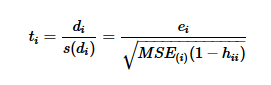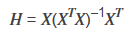Our World Statistics Day conversations have been a great reminder of how much statistics can inform our lives. Do you have an example of how statistics has made a difference in your life? Share your story with the Community!
Choose Language Hide Translation Bar
Highlighted

## REML residuals - Bug or True Value?

Hi,

I am relatively new in building models with REML. I encoutered an usual behavior of residuals and I am not sure if it is a bug or not:

I have no preknowledge regarding the interaction of the different factors, therefore I build a model with stepwise regression (named 'SR: Response - BIC' in the data table) first, where I used the blocking factor as categorical factor. After identifiying the significant parameters, I rebuild the model with REML (named 'REML: Response' in the data table) using the same parameters as in the stepwise regression model, but I changed the blocking factor to a random effect.

After that I saved the studentized residuals of both models. The residuals of the stepwise regression model look quite 'normal'. However, the residuals of the REML model include an huge outlier (run 10), altough the actual vs. predicted plot shows no anomalies. Furthermore, the residual value for run 4 is missing and I can't explain why this is the case.

Does someone know, why ths outlier exists and why the residual is missing? Thanks in advance!

Data description:

• 1 response
• 9 factors
• 1 blocking factor

Software:

• JMP 14.0.0
2 REPLIES 2
Highlighted

## Re: REML residuals - Bug or True Value?

First of all, the choice of a fixed or a random effect depends on the nature of the factor and how you see its contribution to the response. Can you establish the same blocks in the future? Can you use the block level to predict future observations? That sounds like a fixed effect to me. If you cannot establish the same blocks in the future, then it is likely that the levels in this experiment are a sample and the next experiment would be a new sample of the blocks. That sounds like a random effect to me.

Second, the studentized residual is the estimated error in the response (residual) divided by the standard error of the residual. You can see this standard error. Click the red triangle at the top and select Save Columns > Standard Error of Residual. This result is what I found:

(Image removed for confidentiality reasons.)

So the Studentized residual for observation 3 is unusual large because it has an unusually small standard error. (Think t-ratio) The Studentized residual for observation 7 is missing because the standard error (denominator for ratio) is missing.

Learn it once, use it forever!

## Re: REML residuals - Bug or True Value?

Thanks for the answer and your explanation. I do not want to establish the blocks in future, so ‘Block’ is a random effect. I only used the stepwise regression model for variable selection, since this is not implemented with REML.

Let’s assume that following formula is used to calculate the studentized residuals:(https://newonlinecourses.science.psu.edu/stat501/node/401/)

• MSE(i) = mean squared error of residuals, with observation i deleted
• hii = leverage
• ei = yi – yihat (for a model, where observation i is deleted)

I double checked and neither MSE(i), nor ei is 0. I also checked if hii is 1, but this is not the case (https://de.mathworks.com/help/stats/hat-matrix-and-leverage.html). I am really curious how the standard error results in 0 or missing. How exactly is the standard error of residual calculated? Do I miss something here?

Cheers

Article Labels

There are no labels assigned to this post.